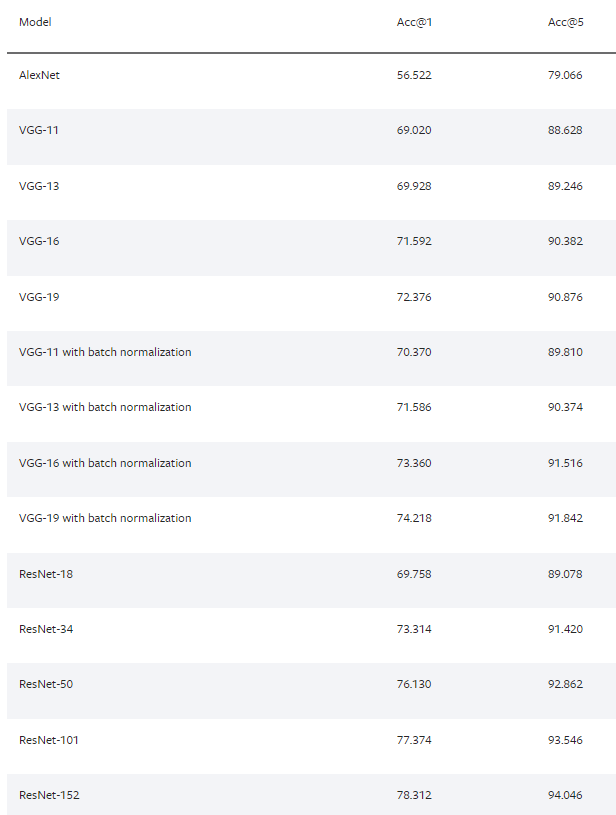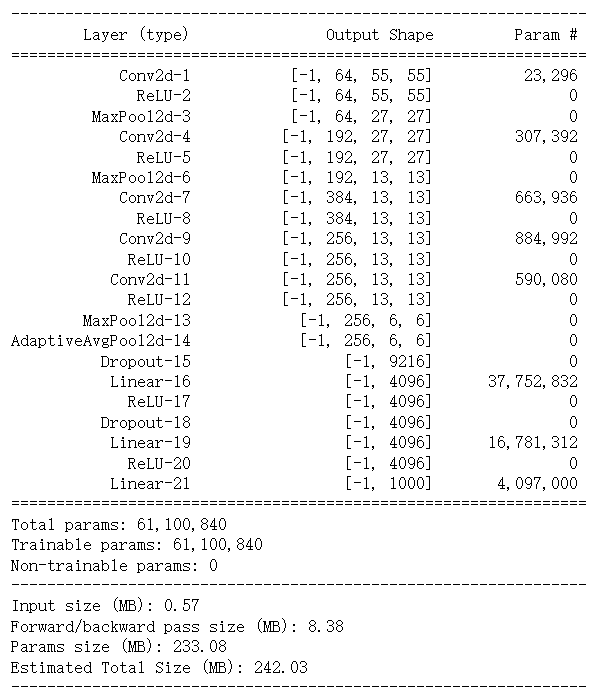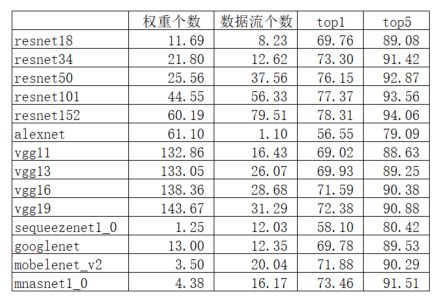# 网络模型统计#https://pytorch.org/vision/stable/models.html
import torchvision.models as models
net = models.alexnet()
#net = models.mnasnet1_0()
net.to('cpu')
#https://github.com/sksq96/pytorch-summary
from torchsummary import summary

summary(net,(3,224,224))• Total params 表示所有超参数(权重)的个数P，每个超参数用浮点数float32表示，占4Byte空间，因此$Params\_size =\frac{P\times4}{1024^2}=\frac{61100840\times4}{1024^2}=233.08MB$
• Forward/backward pass size 表示每层输出的缓存大小，通过列表“Output Shape”计算，最后还要乘以2，保存反向传播的梯度信息：

$$64\times(55^2\times2+27^2)+192\times(27^2\times2+13^2)+384\times13^2\times2\\+256\times(13^2\times4+6^2\times2)+9216+4096\times5+1000\\=1098216$$

Forward/backward pass size:
$$\frac{1098216\times 4\times2}{1024^2}=8.3787MB$$

（其中数据流个数记录的是推理过程中输出层的缓存个数）